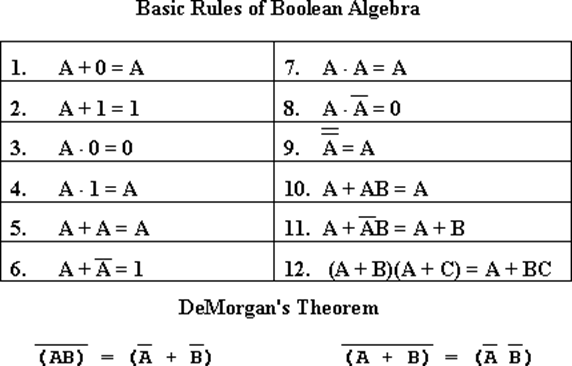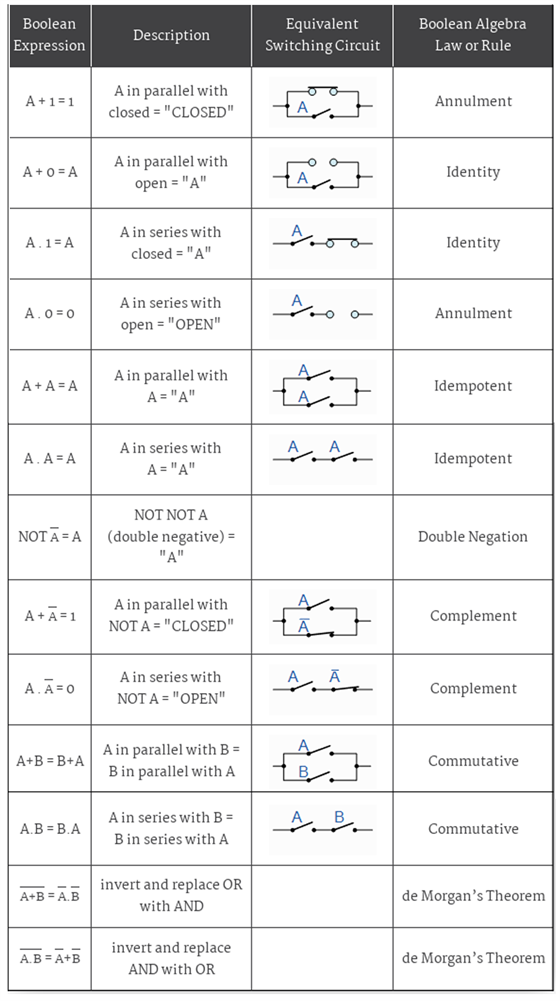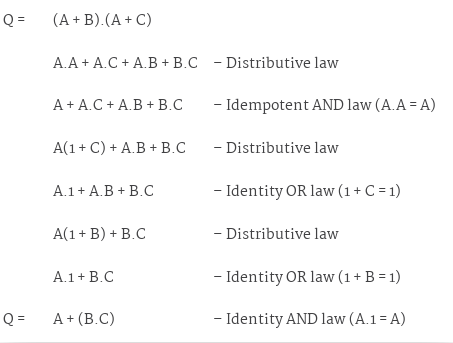Checkout JEE MAINS 2022 Question Paper Analysis : Checkout JEE MAINS 2022 Question Paper Analysis :

# Boolean Algebra Laws

Boolean algebra is one topic where most students get confused. But it is pretty simple if you understand the logic behind it. Boolean algebra is the branch of algebra wherein the values of the variables are either true or false, generally denoted by 1 and 0 respectively. Whereas in elementary algebra we have the values of the variables as numbers and primary operations are Addition and multiplication. Let’s learn Boolean algebra laws in a simpler way.

## Boolean Algebra Rules

Boolean algebra problems can be solved using these Boolean algebra laws. There are few boolean algebra rules to be followed to while solving problems## Applications of Boolean Algebra

Examples of these particular laws of Boolean algebra, rules and theorems for Boolean Algebra are given in the following table.### Solved problems – Using Boolean Algebra Laws

Example:

Using the above laws, we can simplify the given expression:  (A + B)(A + C)You can go ahead and watch videos on BYJU’S – The Learning App to know about boolean algebra calculator, boolean algebra tutorials, and boolean algebra simplification.